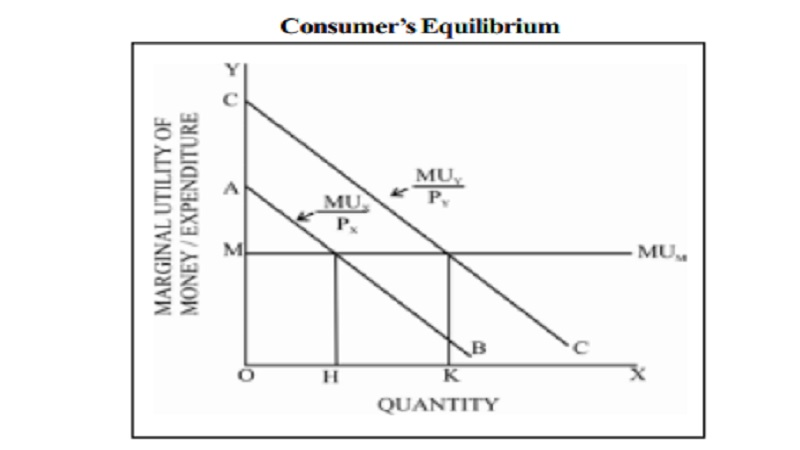Home | | Economics | | Economics | Law Of Equi-Marginal Utility - Definition, Explanation, Importance, Criticism

# Law Of Equi-Marginal Utility - Definition, Explanation, Importance, CriticismIn the words of Prof. Marshall, 'If a person has a thing which can be put to several uses, he will distribute it among these uses in such a way that it has the same marginal utility in all'.

## Law Of Equi-Marginal Utility

The idea of equi-marginal principle was first mentioned by H.H.Gossen (1810-1858) of Germany. Hence it is called Gossen's second Law. Alfred Marshall made significant refinements of this law in his 'Principles of Economics'.

The law of equi-marginal utility explains the behaviour of a consumer when he consumers more than one commodity. Wants are unlimited but the income which is available to the consumers to satisfy all his wants is limited. This law explains how the consumer spends his limited income on various commodities to get maximum satisfaction. The law of equi-marginal utility is also known as the law of substitution or the law of maximum satisfaction or the principle of proportionality between prices and marginal utility.

## Definition

In the words of Prof. Marshall, 'If a person has a thing which can be put to several uses, he will distribute it among these uses in such a way that it has the same marginal utility in all'.

Assumptions

1.        The consumer is rational so he wants to get maximum satisfaction.

2.        The utility of each commodity is measurable.

3.        The marginal utility of money remains constant.

4.        The income of the consumer is given.

5.        The prices of the commodities are given.

6.        The law is based on the law of diminishing marginal utility.

## Explanation of the law

Suppose there are two goods X and Y on which a consumer has to spend a given income. The consumer being rational, he will try to spend his limited income on goods X and Y to maximise his total utility or satisfaction. Only at that point the consumer will be in equilibrium.

According to the law of equi-marginal utility, the consumer will be in equilibrium at the point where the utility derived from the last rupee spent on each is equal.## Limitations of the Law

The law of equi-marginal utility bristles with the following difficulties.

### 1. Indivisibility of Goods

The theory is weakened by the fact that many commodities like a car, a house etc. are indivisible. In the case of indivisible goods, the law is not applicable.

### 2. The Marginal Utility of Money is Not Constant

The theory is based on the assumption that the marginal utility of money is constant. But that is not really so.

3. The Measurement of Utility is not Possible

Marshall states that the price a consumer is willing to pay for a commodity is equal to its marginal utility. But modern economists argue that, if two persons are paying an equal price for given commodity, it does not mean that both are getting the same level of utility. Thus utility is a subjective concept, which cannot be measured, in quantitative terms.

### 4. Utilities are Interdependent

This law assumes that commodities are independent and therefore their marginal utilities are also independent. But in real life commodities are either substitutes or complements. Their utilities are therefore interdependent.

### 5. Indefinite Budget Period

According to Prof. K.E. Boulding, indefinite budget period is another difficulty in the law. Normally the budget period is assumed to be a year. But there are certain commodities which are available in several succeeding accounting periods. It is difficult to calculate marginal utility for such commodities.

In conclusion, we may say all prudent and rational persons are expected to act upon the law consciously or unconsciously. As Chapman puts it,

'We are not, of course compelled to distribute our incomes according to the law of substitution or equi-marginal expenditure, as a stone thrown into the air is compelled, in a sense to fall back to the earth, but as a matter of fact, we do in a certain rough fashion, because we are reasonable.'

## Importance

According to Marshall, 'the applications of this principle extend over almost every field of economic activity.'

### 1. It applies to consumption

Every rational human being wants to get maximum satisfaction with his limited means. The consumer arranges his expenditure in such a way that,   M U x/Px    =       M U y/Py    =          M Uz/ P z    so that he will get maximum satisfaction.

### 2. It applies to production

The aim of the producer is to get maximum output with least-cost, so that his profit will be maximum. Towards this end, he will substitute one factor for another till

MPl / Pl = MPc / Pc = MPn / Pn

### 3. Distribution of Earnings Between Savings and Consumption

According to Marshall, a prudent person will endeavour to distribute his resources between his present needs and future needs in such a way that the marginal utility of the last rupee put in savings is equal to the marginal utility of the last rupee spent on consumption.

### 4. It applies to distribution

The general theory of distribution involves the principle of substitution. In distribution, the rewards to the various factors of production, that is their relative shares, are determined by the principle of equi-marginal utility.

### 5. It Applies to Public Finance

The principle of 'Maximum Social Advantage' as enunciated by Professors Hicks and Dalton states that, the revenue should be distributed in such a way that the last unit of expenditure on various programmes brings equal welfare, so that social welfare is maximised.

### 6. Expenditure of Time

Prof. Boulding relates Marshall's law of equi-marginal utility to the expenditures of limited time, i.e. twenty-four hours. He states that a person should spend his limited time among alternative uses such as reading; studying and gardening, in such a way that the marginal utility from all these uses are equal.

Study Material, Lecturing Notes, Assignment, Reference, Wiki description explanation, brief detail

Related Topics